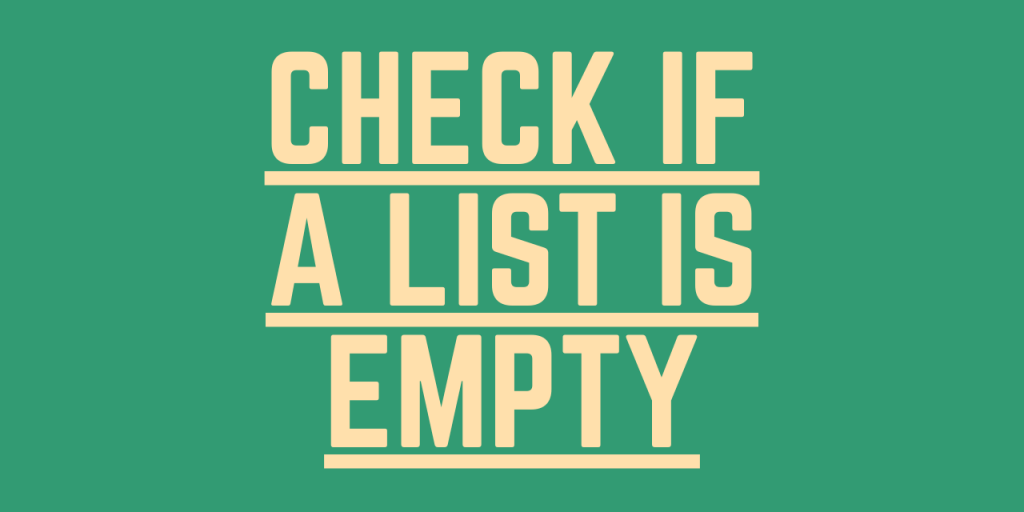# Check if a List is Empty – 3 Easy MethodsHey folks! Hope you all are doing well. In this article, we will be focusing on the Different techniques to check if a list is empty.

Before getting into it, let us have a look at Python List.

## What is a Python List?

Python List is a data structure that stores data dynamically into it. In Python, it serves the purpose of Arrays. Moreover, Lists can store heterogeneous elements i.e. elements of different data types together into it.

Now, having understood the working of a list, let us now understand different methods to check whether a list is empty or not.

## Technique 1: Using len() function

`Python len() function `can be used to check if a list is empty. If the len() function returns zero, the list is said to be empty.

Example:

```lst = [] ## empty list

length = len(lst)

if length == 0:
print("List is empty -- ",length)
else:
print("List isn't empty -- ",length)
```

Output:

```List is empty --  0
```

## Technique 2: Using a conditional statement

Python Conditional if statement can be used to check whether the list is empty or not as shown below–

Syntax:

```if not list:
#empty
else:

```

Example:

```lst = [] ## empty list

if not lst:
print("List is empty.")
else:
print("List isn't empty.")
```

In the above example, we have used if statement to validate for the presence of any element in the list.

Output:

```List is empty.
```

## Technique 3: Direct Comparison

We can check for the presence of an empty list by directly comparing the list with an empty list i.e. [ ] as shown below–

Syntax:

```if list == []:
#empty
else:

```

Example:

```lst = list() ## empty list

if lst == []:
print("List is empty.")
else:
print("List isn't empty.")
```

Here, we have compared the specified list with an empty list to check whether the given list is empty or not.

Output:

```List is empty.
```

## Conclusion

By this, we have come to the end of this topic. Feel free to comment below in case you come across any questions.

Till then, Happy Learning!!